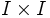# Three sides lemma

Jump to: navigation, search

This term is nonstandard and is being used locally within the wiki. For its use outside the wiki, please define the term when using it.

This article is about the statement of a simple but indispensable lemma in topology

## Statement

The inclusion of three sides in the unit square is equivalent to the inclusion of one side in the unit square, viz., is equivalent to the inclusion of a boundary$I$ in$I \times I$.

An explicit isotopy can also be written down, which is useful in some situations.

## Applications

The crux of the application of the three sides lemma is that it allows us to extend homotopies given both initial conditions and final conditions. The homotopy to be extended here is the middle side, and the initial and final conditions are the extreme sides.

• The three sides lemma is sometimes used to give a different definition of the second homotopy group of a pair. In fact, the generalized three sides lemma is used to give alternative definitions for all the higher homotopy groups.
• The three sides lemma gives a quick proof that the inclusion of the two endpoints in the unit interval is a cofibration (contrast this with the two sides lemma, which shows that the inclusion of one endpoint is a cofibration). An explicit retraction can also be written down.
• The three sides lemma gives a proof that the inclusion of the disjoint union of the center and boundary sphere of a disc, in the disc, is a cofibration. This is used to prove that the inclusion of a point in a manifold is a cofibration Further information: Center plus boundary in disc is cofibration
• The three sides lemma is also used in proofs involving fibrations (or more generally, Serre fibrations). For instance, it shows that for a Serre fibration, the fibers at two points in the same path component are homotopy-equivalent. Further information: Fibers are homotopy-equivalent for Serre fibrations